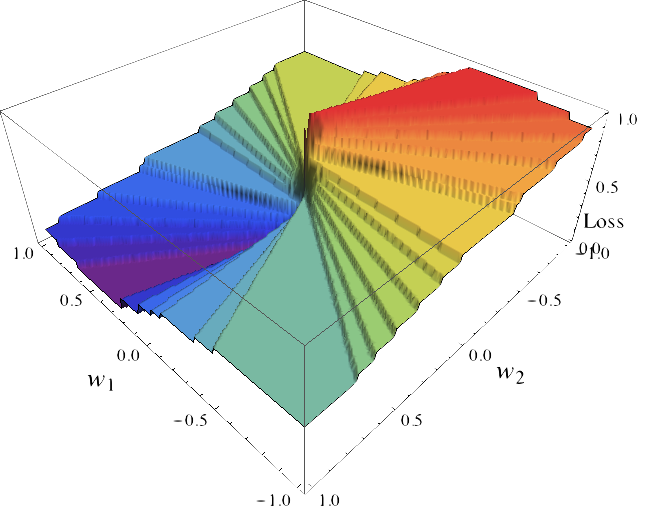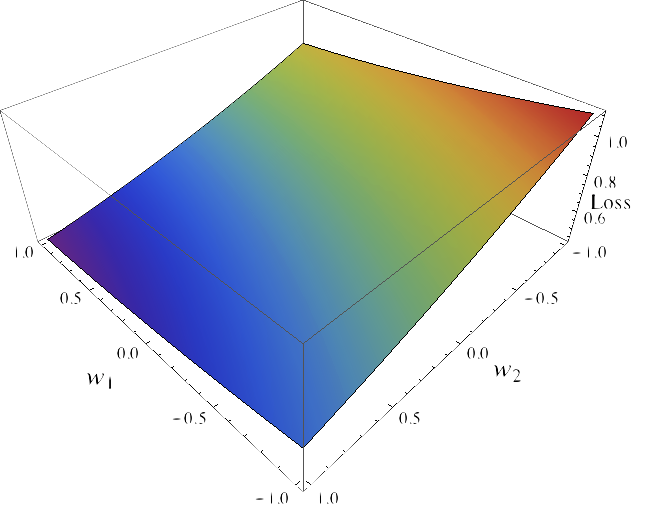# fa.bianp.net

TL; DR These are some notes on calibration of surrogate loss functions in the context of machine learning. But mostly it is an excuse to post some images I made.

In the binary-class classification setting we are given $n$ training samples $\{(X_1, Y_1), \ldots, (X_n, Y_n)\}$, where $X_i$ belongs to some sample space $\mathcal{X}$, usually $\mathbb{R}^p$ but for the purpose of this post we can keep i abstract, and $y_i \in \{-1, 1\}$ is an integer representing the class label.

We are also given a loss function $\ell: \{-1, 1\} \times \{-1, 1\} \to \mathbb{R}$ that measures the error of a given prediction. The value of the loss function $\ell$ at an arbitrary point $(y, \hat{y})$ is interpreted as the cost incurred by predicting $\hat{y}$ when the true label is $y$. In classification this function is often the zero-one loss, that is, $\ell(y, \hat{y})$ is zero when $y = \hat{y}$ and one otherwise.

The goal is to find a function $h: \mathcal{X} \to [k]$, the classifier, with the smallest expected loss on a new sample. In other words, we seek to find a function $h$ that minimizes the expected $\ell$-risk, given by $$\mathcal{R}_{\ell}(h) = \mathbb{E}_{X \times Y}[\ell(Y, h(X))]$$

In theory, we could directly minimize the $\ell$-risk and we would have the optimal classifier, also known as Bayes predictor. However, there are several problems associated with this approach. One is that the probability distribution of $X \times Y$ is unknown, thus computing the exact expected value is not feasible. It must be approximated by the empirical risk. Another issue is that this quantity is difficult to optimize because the function $\ell$ is discontinuous. Take for example a problem in which $\mathcal{X} = \mathbb{R}^2, k=2$, and we seek to find the linear function $f(X) = \text{sign}(X w), w \in \mathbb{R}^2$ and that minimizes the $\ell$-risk. As a function of the parameter $w$ this function looks something likeThis function is discontinuous with large, flat regions and is thus extremely hard to optimize using gradient-based methods. For this reason it is usual to consider a proxy to the loss called a surrogate loss function. For computational reasons this is usually convex function $\Psi: \mathbb{R} \to \mathbb{R}_+$. An example of such surrogate loss functions is the hinge loss, $\Psi(t) = \max(1-t, 0)$, which is the loss used by Support Vector Machines (SVMs). Another example is the logistic loss, $\Psi(t) = 1/(1 + \exp(-t))$, used by the logistic regression model. If we consider the logistic loss, minimizing the $\Psi$-risk, given by $\mathbb{E}_{X \times Y}[\Psi(Y, f(X))]$, of the function $f(X) = X w$ becomes a much more more tractable optimization problem:In short, we have replaced the $\ell$-risk which is computationally difficult to optimize with the $\Psi$-risk which has more advantageous properties. A natural questions to ask is how much have we lost by this change. The property of whether minimizing the $\Psi$-risk leads to a function that also minimizes the $\ell$-risk is often referred to as consistency or calibration. For a more formal definition see  and . This property will depend on the surrogate function $\Psi$: for some functions $\Psi$ it will be verified the consistency property and for some not. One of the most useful characterizations was given in  and states that if $\Psi$ is convex then it is consistent if and only if it is differentiable at zero and $\Psi'(0) < 0$. This includes most of the commonly used surrogate loss functions, including hinge, logistic regression and Huber loss functions.1. P. L. Bartlett, M. I. Jordan, and J. D. McAuliffe, “Convexity , Classification , and Risk Bounds,” J. Am. Stat. Assoc., pp. 1–36, 2003.

2. A. Tewari and P. L. Bartlett, “On the Consistency of Multiclass Classification Methods,” J. Mach. Learn. Res., vol. 8, pp. 1007–1025, 2007.